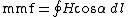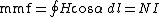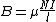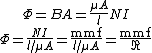# reluctance

(redirected from reluctances)
Also found in: Dictionary, Thesaurus.

## reluctance

(less commonly), reluctancy
Physics a measure of the resistance of a closed magnetic circuit to a magnetic flux, equal to the ratio of the magnetomotive force to the magnetic flux
Collins Discovery Encyclopedia, 1st edition © HarperCollins Publishers 2005

## Reluctance

A property of a magnetic circuit analogous to resistance in an electric circuit.

Every line of magnetic flux is a closed path. Whenever the flux is largely confined to a well-defined closed path, there is a magnetic circuit. That part of the flux that departs from the path is called flux leakage.

For any closed path of length l in a magnetic field H, the line integral of H cos α dl around the path is the magnetomotive force (mmf) of the path, as in

(1)Eq. (1), where α is the angle between H and the path. If the path encloses N conductors, each with current I, Eq. (2) holds.
(2)Consider the closely wound toroid shown in the illustration. For this arrangement of currents, the magnetic field is almost entirely within the toroidal coil, and there the flux density or magnetic induction B is given by

(3)Eq. (3), where l is the mean circumference of the toroid and μ is the permeability. The flux Φ within the toroid of cross-sectional area A is given by either form of
(4)Eqs. (4), which is similar in form to the equation for the electric circuit, although nothing actually flows in the magnetic circuit. The factor l/μA is called the reluctance of the magnetic circuit. The reluctance is not constant because the permeability μ varies with changing flux density.

McGraw-Hill Concise Encyclopedia of Physics. © 2002 by The McGraw-Hill Companies, Inc.
The following article is from The Great Soviet Encyclopedia (1979). It might be outdated or ideologically biased.

## Reluctance

a characteristic of a magnetic circuit; the reluctance R m is equal to the ratio of the magnetomotive force F acting on a magnetic circuit to the magnetic flux Φ developed in the circuit. For a uniform section of a magnetic circuit the reluctance can be calculated from the formula Rm = l/μμ0S, where l and S are the length and cross section of the section of the magnetic circuit, respectively; μ is the relative magnetic permeability of the material in the circuit; and μ0 is the magnetic constant.

In the case of a nonuniform magnetic circuit composed of successive uniform sections having different values of l, S, and μ, the reluctance is equal to the sum of the values of Rm for the uniform sections. Such a computation is approximate, since the formula does not take into account “magnetic leakage” (the diffusion of magnetic flux into the space surrounding the magnetic circuit), the nonuniformity of the magnetic field in the circuit, and the nonlinear dependence of the reluctance on the field. In a variable magnetic field the reluctance is a complex quantity, because in this case μ, is a function of the frequency of the electromagnetic oscillations. The unit of reluctance in the International System of Units is the ampere or ampere-turn per weber (A/Wb), and in the cgs system of units, the gilbert per maxwell (Gb/Mx); 1 A/Wb = 4 × 10-9 Gb/Mx ≈ 1.2566 × 10-8 Gb/Mx.

## reluctance

[ri′lək·təns]
(electromagnetism)
A measure of the opposition presented to magnetic flux in a magnetic circuit, analogous to resistance in an electric circuit; it is equal to magnetomotive force divided by magnetic flux. Also known as magnetic reluctance.
McGraw-Hill Dictionary of Scientific & Technical Terms, 6E, Copyright © 2003 by The McGraw-Hill Companies, Inc.
References in periodicals archive ?
, the reluctance of the soft magnetic materials was included to consider the nonlinear magnetization characteristic, but the most important B-H magnetizing curve was determined based on B-H experimental data by a trial and error method for calculating the reluctance of soft magnetic materials.
Some electromagnetic mathematical models use an electromagnetic force fitting formula based on experimental data, some models do not consider the reluctance of the soft magnetic materials, and some only took segmental magnetic properties of the magnetic materials into account.
For the HSV, the functional relationship between the total magnetic flux and the total reluctance of the magnetic circuit is described as
where O is the total magnetic flux; N is the coil turns; I is the current; .Rtotai is the total reluctance of magnetic circuit.
To accurately describe the nonlinear magnetization and magnetic saturation of the HSV, the mathematical model should take the reluctance of the iron core and armature soft magnetic materials into consideration.
where [R.sub.gap1] is the gap reluctance between main pole and armature; [R.sub.gap2] is the gap reluctance between side pole and armature; [R.sub.arm] is the reluctance of armature; [R.sub.iron] is the reluctance of iron core.
On the one hand, the reluctance of the soft magnetic material has an effect on the electromagnetic conversion, and, on the other hand, the drive current has an effect on the reluctance.
This is because the electromagnetic force of the HSV is inversely proportional to the reluctance under a constant drive current and coil turns based on (1)-(4) and (9).

Site: Follow: Share:
Open / Close